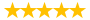# PROJ586 W4 Homework Crashing and Earned Value Problems

Question # 00003567 Posted By: neil2103 Updated on: 11/15/2013 08:06 AM Due on: 11/27/2013
Subject Gender Studies Tutorials:
Question

In this homework assignment, you will be working through two crashing problems and four earned value problems. All the information you need is included within this Homework packet. Make sure you answer all parts of the questions in their entirety.

Once you have worked through the problems, please post your responses to the Drop Box. Please refer to the course syllabus for the due date.

PROBLEM 1

Use the network diagram below and the additional information provided to answer the corresponding questions. [15 points]a) The crash cost per day per activity. [10 points]

b) Which activities should be crashed to meet a project deadline of 10 days at minimum cost? What is the cost impact of crashing these activities? [3 points]

c) Find the new budget (or cost of the project). [2 points]

PROBLEM 2

Use the network diagram below and the additional information provided to answer the corresponding questions. [13 points]a) The crash cost per day per activity. [10 points]

b) Which activities should be crashed to meet a project deadline of 13 days at minimum cost? What is the cost impact of crashing these activities? [3 points]

PROBLEM 3 [8 points]

 Month ACWP BCWS BCWP 22 \$540 \$523 \$535

a) Calculate the CV (Cost Variance). [2 points]

b) Is the CV over or under budget? [2 points]

c) Calculate the SV (Schedule Variance). [2 points]

d) Is the SV ahead or behind schedule? [2 points]

PROBLEM 4 [8 points]

 Month ACWP BCWS BCWP 5 \$34 \$42 \$39

a) Calculate the CPI (Cost Performance Index). [2 points]

b) Is the project cost efficient or not efficient? [2 point]

c) Calculate the SPI (Schedule Performance Index) [2 points]

d) Is the schedule efficient or not efficient? [2 point]

PROBLEM 5 [8 points]

 Day ACWP BCWS BCWP 65 \$550 \$735 \$678

a) Calculate the CV (Cost Variance). [2 points]

b) Calculate the CPI (Cost Performance Index). [2 points]

c) Calculate the SV (Schedule Variance). [2 points]

d) Calculate the SPI (Schedule Performance Index) [2 points]

PROBLEM 6 [8 points]

 Day ACWP BCWS BCWP 65 \$750 \$735 \$678

a) Calculate the CV (Cost Variance). [1 point]

b) Calculate the CPI (Cost Performance Index). [1 point]

c) Calculate the SV (Schedule Variance). [1 point]

d) Calculate the SPI (Schedule Performance Index) [1 points]

e) Explain the project performance in terms of efficiency, budget and schedule. What should the project manager do for the project? [4 points]

Attachments
Tutorials for this Question
1. ## Solution: PROJ586 W4 Homework Crashing and Earned Value Problems

Tutorial # 00003372 Posted By: neil2103 Posted on: 11/15/2013 08:07 AM
Puchased By: 13
Tutorial Preview
The solution of PROJ586 W4 Homework Crashing and Earned Value Problems...
Attachments
PROJ586_W4_Homework_EV_Solutions.docx (62.62 KB)
Recent Feedback
Rated By Feedback Comments Rated On
Mor...naBeThe work is of top and best quality 10/29/2018
rm...h4Well-organized and best work provided 08/31/2017
ad...rilReally appreciable tutorial service 10/24/2016
u...19Provide the work at reasonable prices 10/28/2015
dm...n4Far better than classroom learning 10/23/2015

Great! We have found the solution of this question!